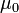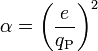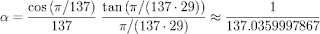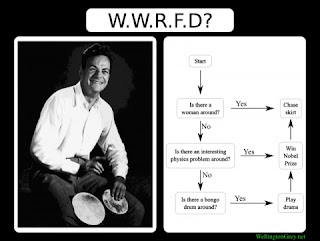## Tuesday, May 19, 2009

### Fine Structure Constant alpha = 1/137

From the Wiki entry on The Fine-Structure Constant:

As a dimensionless constant which does not seem to be directly related to any mathematical constant, the fine-structure constant has long fascinated physicists. Richard Feynman, one of the founders of quantum electrodynamics, referred to it in these terms:

“ It has been a mystery ever since it was discovered more than fifty years ago, and all good theoretical physicists put this number up on their wall and worry about it. Immediately you would like to know where this number for a coupling comes from: is it related to π or perhaps to the base of natural logarithms? Nobody knows. It's one of the greatest damn mysteries of physics: a magic number that comes to us with no understanding by man. You might say the 'hand of God' wrote that number, and 'we don't know how He pushed his pencil.' We know what kind of a dance to do experimentally to measure this number very accurately, but we don't know what kind of dance to do on the computer to make this number come out, without putting it in secretly! ”

—Richard P. Feynman (1985), QED: The Strange Theory of Light and Matter, Princeton University Press, p. 129, ISBN 0691083886

Three standard and equivalent definitions of α in terms of other fundamental physical constants are:where:is the elementary charge;is the reduced Planck constant;is the speed of light in vacuum;is the electric constant;is the magnetic constant or vacuum permeability, a defined conversion factor;is the constant of Coulomb's law.

The fine structure constant α has several physical interpretations.

In electrostatic cgs units, the unit of electric charge, the statcoulomb, is defined so that the permittivity factor, 4πε0, is 1 and dimensionless. The fine-structure constant then becomesPHYSICAL INTERPRETATIONS

* The square of the ratio of the elementary charge to the Planck charge* The ratio of two energies: (i) the energy needed to overcome the electrostatic repulsion between two electrons when the distance between them is reduced from infinity to some finite d, and (ii) the energy of a single photon of wavelength λ = 2πd (see Planck relation):* The ratio of the velocity of the electron in the Bohr model of the atom to the speed of light. Hence the square of α is the ratio between the electron rest mass (511 keV) and the Hartree energy (27.2 eV = 2 Ry).

* In quantum electrodynamics, α is the coupling constant determining the strength of the interaction between electrons and photons. The theory does not predict its value; therefore it must be determined experimentally. In fact, α is one of the ~20 empirical parameters in the Standard Model of particle physics, whose value is not determined within the Standard Model.

* In the electroweak theory unifying the weak interaction with electromagnetism, α is absorbed into two other coupling constants associated with the electroweak gauge fields. In this theory, the electromagnetic interaction is treated as a mixture of interactions associated with the electroweak fields. The strength of the electromagnetic interaction varies with the strength of the energy field.

When perturbation theory is applied to quantum electrodynamics, the resulting perturbative expansions for physical results are expressed as power series in α. Because α is clearly less than 1, higher powers of α are soon unimportant, making perturbation theory practical. In contrast, the large value of the corresponding factors in quantum chromodynamics makes calculations involving the strong force extremely difficult.

According to the theory of the renormalization group, the value of the fine structure constant (the strength of the electromagnetic interaction) grows logarithmically as the energy scale is increased. The observed value of α is associated with the energy scale of the electron mass; the electron is a lower bound for this energy scale because it (and the positron) is the lightest charged object whose quantum loops can contribute to the running. Therefore 1/137.036 is the value of the fine structure constant at zero energy. Moreover, as the energy scale increases, the strength of the electromagnetic interaction approaches that of the other two fundamental interactions, a fact important for grand unification theories. If quantum electrodynamics were an exact theory, the fine structure constant would actually diverge at an energy known as the Landau pole. This fact makes quantum electrodynamics inconsistent beyond the perturbative expansions.

The mathematician James Gilson has suggested  that the fine-structure constant has the value:29 and 137 being the 10th and 33rd prime numbers. The difference between the 2007 CODATA value for α and this theoretical value is about 3 x 10^-11, about 6 times the standard error for the measured value.Anonymous said...

All those "physical explanations" are not really physical: the corresponding ratios do not arise naturally in course of calculations. That is why alpha was "discovered" as a fine structure constant (a relativistic effect in a non relativistic theory).

I have a simple mechanical model where a dimensionless coupling constant is expressed via the ratio of masses of constituents of a compound system. That can be called a natural physical explanation.

Unknown said...

Hi there, pages 8 10 and 11 may be of interest to you related to the solfeggio scale, prime cross and I Ching. Pyramids water and photons.

Many thanks for the inspiration

https://www.scribd.com/document/324851878/Absolute-Manifestation-Expression-the-Ocean-Lives-Within-the-Drop

http://pdfsr.com/pdf/absolutemanifestationexpressiontheoceanliveswithinthedrop.pdf

in case the previous link does not work. =-)

Borei said...

https://finestructureconstantspeedoflightusdbill.yolasite.com/

Fine structure constant, Speed of light, encoded on the USD Bill.
Peace.